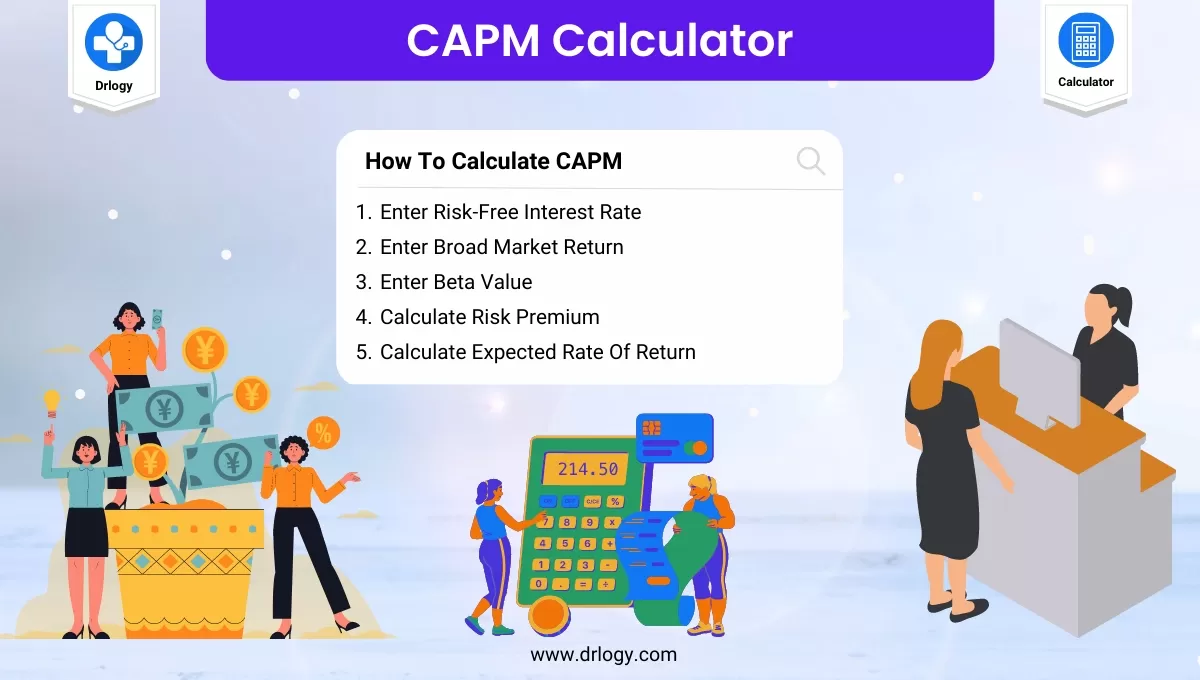• Health
• Pet
• Unit Conversion
• Medical# Capital Asset Pricing Model (CAPM) Calculator

## CAPM Calculator

###### Result

The CAPM calculator (capital asset pricing model) aims to determine the expected return of a particular asset or investment.

## CAPM Calculator Overview

• The Capital Asset Pricing Model (CAPM) is a financial model used to calculate the expected return on an investment based on its level of risk.
• A CAPM calculator is a tool that helps investors determine the expected return on a stock or portfolio of stocks, based on the risk-free rate, market risk premium, and beta of the stock or portfolio.
• The CAPM is widely used in finance as a tool for evaluating the relative attractiveness of different investments and for setting the required rate of return for an investment based on its level of risk.

## What is CAPM?

• The Capital Asset Pricing Model (CAPM) is a financial model used to calculate the expected return on an investment based on its level of risk.
• CAPM assumes that investors are risk-averse and require compensation for taking on additional risk.
• CAPM considers the risk-free rate, market risk premium, and the beta of an asset to determine its expected return.
• The risk-free rate is the rate of return on a risk-free investment, such as a government bond.
• The market risk premium is the expected excess return of the overall market over the risk-free rate.
• Beta measures the volatility of an asset in relation to the overall market.
• CAPM helps investors evaluate the relative attractiveness of different investments and set the required rate of return for an investment based on its level of risk.

## Steps To Calculate CAPM

Here are the steps to calculate CAPM.

• Enter Risk-Free Interest Rate
• Enter Beta Value
• Calculate the Expected Rate Of Return

## CAPM Formula

Here are formula to calculate CAPM.

• Market Risk Premium = Rm - Rf

where:

• R – Expected rate of return of an asset or investment;
• Rf – Risk-free interest rate, typically taken as the yield on a long-term government bond in the country where the project is based;
• Rm – Broad market return; and
• Beta – Parameter of the market risk.

## How To Calculate CAPM

Here's how to calculate CAPM with an example using the formula:

The CAPM formula is:

Expected Return = Risk-Free Rate + Beta x (Market Risk Premium)

• Let's assume that the risk-free rate is 2%, the expected return of the market is 8%, and the beta of a stock is 1.2.
• First, we need to calculate the market risk premium:

Market Risk Premium = Rm - Rf

Market Risk Premium = 8% - 2%

• Next, we can calculate the risk premium for the stock:

Risk Premium = 1.2 x (6%) = 7.2%

Finally, we can calculate the expected return of the stock:

Expected Return = Risk-Free Rate + Risk Premium

Expected Return = 2% + 7.2% = 9.2%

Therefore, according to CAPM, the expected return on the stock with a beta of 1.2 is 9.2%.

• If an investor requires a return higher than 9.2% to compensate for the risk of the investment, the stock would not be considered an attractive investment according to CAPM.
• However, if the expected return of the stock is higher than 9.2%, the investor may consider the stock to be an attractive investment based on the level of risk involved.

## CAPM Calculator Benefits

Here are some benefits of using a CAPM calculator:

1. Helps in making informed investment decisions: CAPM calculator provides an estimate of the expected return on an investment based on its level of risk, which can help investors make informed decisions about which investments to choose.
2. Enables efficient portfolio construction: CAPM calculator can help investors construct efficient portfolios by identifying assets that offer the best risk-return tradeoff.
3. Provides a benchmark for evaluating investment performance: CAPM can be used as a benchmark to evaluate the performance of an investment or portfolio over time. If the actual return is significantly different from the expected return, it may indicate a problem with the investment strategy or the portfolio construction.
4. Helps in determining the required rate of return: CAPM calculator helps in determining the required rate of return for an investment based on its level of risk. This can help investors decide whether an investment is worth making or not.
5. Widely used in finance: CAPM is widely used in finance as a tool for evaluating the relative attractiveness of different investments and for setting the required rate of return for an investment based on its level of risk.

Summary

Overall, CAPM calculator is a valuable tool that helps investors estimate the expected return on an investment based on its level of risk, allowing for informed investment decisions. Check More Finance  Related Calculator on Drlogy Calculator to get exact business and financial solutions for growth.

Reference

• Capital asset pricing model - Wikipedia .
• What Is the Capital Asset Pricing Model (CAPM)? .
favorite_border 1047 Likes

View More Health

## Medical Calculator

View More Medical

View More Pet

## Unit Conversion Calculator

View More Unit Conversion

## Financial Calculator

View More Financial
Drlogy®
The Power To Health